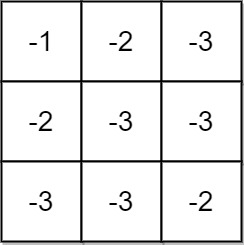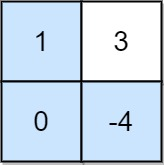1594. Maximum Non Negative Product in a Matrix
Medium
730
36

You are given a `m x n` matrix `grid`. Initially, you are located at the top-left corner `(0, 0)`, and in each step, you can only move right or down in the matrix.

Among all possible paths starting from the top-left corner `(0, 0)` and ending in the bottom-right corner `(m - 1, n - 1)`, find the path with the maximum non-negative product. The product of a path is the product of all integers in the grid cells visited along the path.

Return the maximum non-negative product modulo `109 + 7`. If the maximum product is negative, return `-1`.

Notice that the modulo is performed after getting the maximum product.

Example 1:```Input: grid = [[-1,-2,-3],[-2,-3,-3],[-3,-3,-2]]
Output: -1
Explanation: It is not possible to get non-negative product in the path from (0, 0) to (2, 2), so return -1.
```

Example 2:```Input: grid = [[1,-2,1],[1,-2,1],[3,-4,1]]
Output: 8
Explanation: Maximum non-negative product is shown (1 * 1 * -2 * -4 * 1 = 8).
```

Example 3:```Input: grid = [[1,3],[0,-4]]
Output: 0
Explanation: Maximum non-negative product is shown (1 * 0 * -4 = 0).
```

Constraints:

• `m == grid.length`
• `n == grid[i].length`
• `1 <= m, n <= 15`
• `-4 <= grid[i][j] <= 4`
Accepted
20.8K
Submissions
62.8K
Acceptance Rate
33.1%

Seen this question in a real interview before?
1/4
Yes
No

Discussion (0)

Related Topics